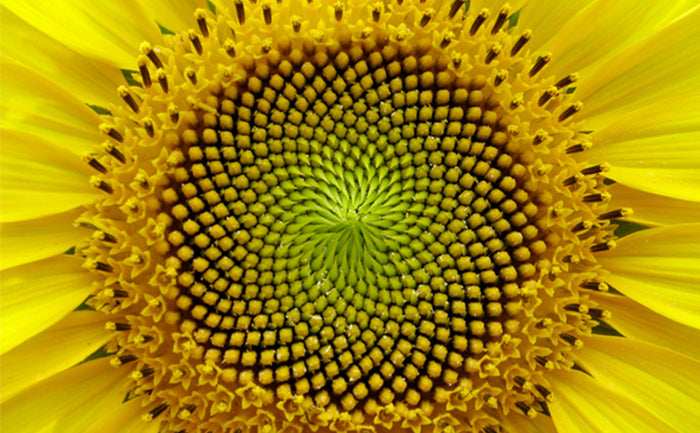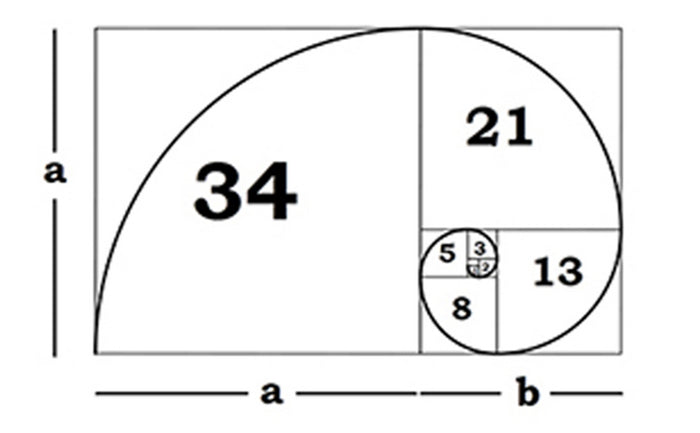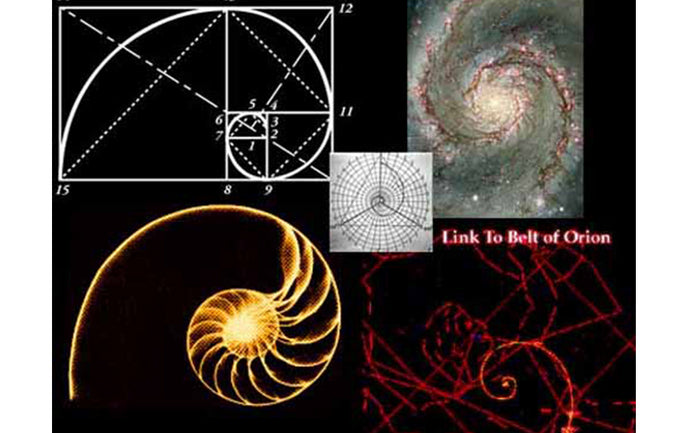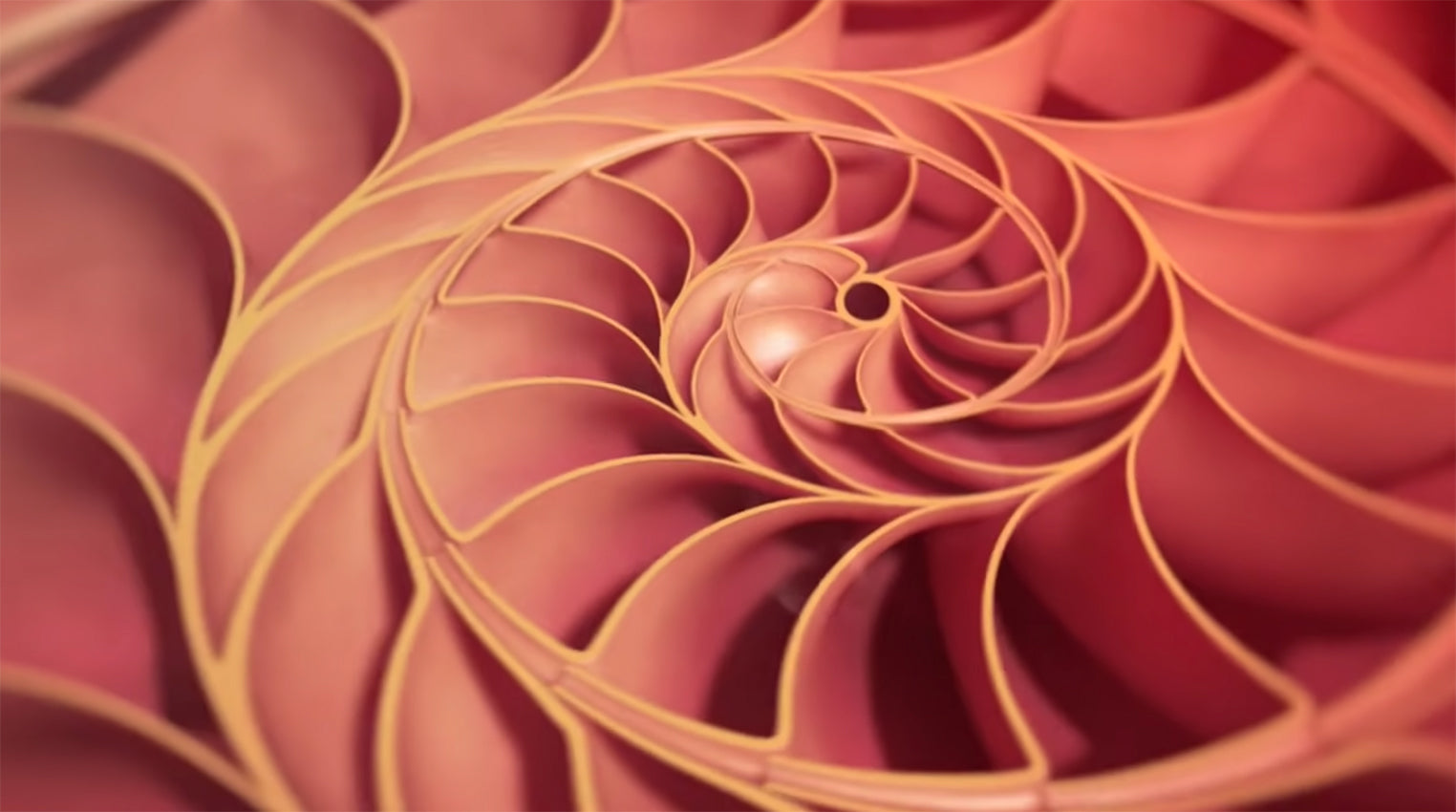# The Mysteries Of The Universe - The Fibonacci Sequence

The Universe is full o mysteries, and we have yet to discover and explore many of them. Today, we shall explore the sacred "Fibonacci sequence".

So, what exactly is the so-called sequence of Fibonacci, the famous mathematician of the Middle Ages, born in Pisa (Italy)? And what secrets does it hide?

## The Fibonacci Sequence

The Fibonacci sequence is probably the most common relationship that occurs in nature. We can see it in the shells of snails, the arrangement of sunflower seeds on the flower cap, in the pattern found on the wings of dragonflies, in the arrangement of hexagons in the honeycomb of bees, as well as in the shape of the Milky Way or other galaxies.The string is made up of a sequence of numbers called: 0,1,1,2,3,5,8,13,21,34,55,89,144... each number being equal to the sum of the numbers before it and the difference of the next two numbers. For example 0+1=1; 1+1=2; 1+2=3 etc, and 144-89=55; 89-55=34; 55-34=21 etc. This string continues to infinity.

The mathematical description of the infinite Fibonacci string may seem hard to understand, but if you look at this animation video you will immediately understand what it is all about.

Related to the Fibonacci string is the Golden Ratio (also called the Golden Section, or the Divine Section), which has applications in art and architecture, mathematics, and even financial systems. The ratio of any Fibonacci number to its predecessor is 1.618, or phi (ϕ).

## Divine or Golden Section, Golden Ratio

The Greek letter ϕ phi symbolizes the Divine Section, this ϕ is more easily visualized in geometric form and is represented by a rectangle formed by joining a square and a rectangle whose ratio is 1 to 1.618 to the square. This operation can be repeated in both directions ad infinitum.

If we draw a quarter circle in each square so that the line joining the centers goes through the touching point to give a smooth curve we obtain the Golden Spiral (or Divine Spiral). For an art student, this is nothing new, as it has been taught since the time of Leonardo Da Vinci as a standard for creating balanced and harmonious compositions. Less well-known is the fact that the Golden Spiral is the simplest natural mathematical pattern. In this pattern, snail shells, shells, animal horns, flowers, plants, etc. are inscribed. People use figures only to organize information.

The golden ratio can be applied to any geometric shape, including circles, triangles, pyramids, or prisms, as demonstrated in the animated film. The Golden Ratio is made up of thirds and sixths. It is the connection between 2 and 3, including the relationship between any even and odd number. The proportion itself is the relationship between numbers, but our understanding of the world we live in is not based on numbers, but on what those numbers represent.An eloquent example is the arrangement of sunflower seeds. They are arranged in the pattern of the Golden Spiral. Such an arrangement gives the flower a biological advantage by maximizing the number of seeds that can fit on the flower cap.

If we draw a graph for any number system, we get a pattern or template. The Divine Proportion is not the only mathematical pattern that tends towards infinity, there are many more such patterns. Knowing this, ask yourself the question: how could infinity repeat itself? How could it happen twice? Infinity represents what is eternal, what is truly whole.